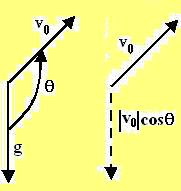## Wednesday, July 18, 2007

### Irodov Problem 1.28(a) Let g be the acceleration vector of the body due to gravitation, then in vector notation, the displacement of the body with respect to time is simply given by, r(t) = v0t + ½ gt2.
(b) The mean velocity vector is
simply displacement over time r(t)/t = v0 + ½ gt (1).
As seen in the figure, the cosine of the angle between the vector g and the vector v0 , is given by,Note that the cosine may be a negative quantity since V0 is actually directed above the horizontal in the diagram while g is pointing downwards.
The component of v0 along the direction of g is then given byNote that this value ofitself will be negative since the cosine is negative but it will directed towards g, in other words the negative value will indicate that it is directed away from g.

The component of velocity in the direction of g will be become zero when,The small object will take double this time to reach back to the horizontal, thus, the total time the body will travel is.

Now, knowing the total time the body traveled, we can use (1) to calculate the value of as,